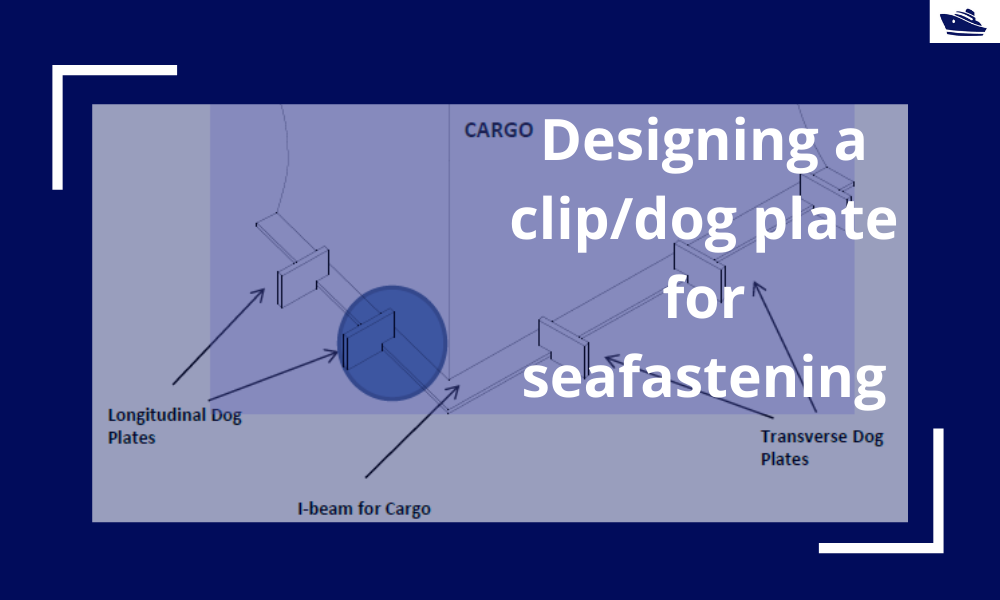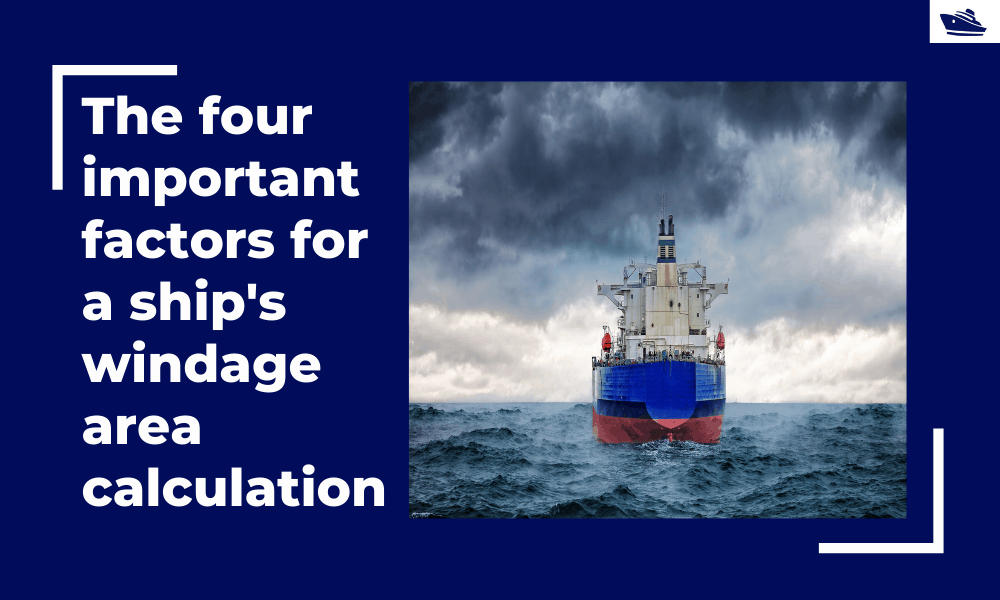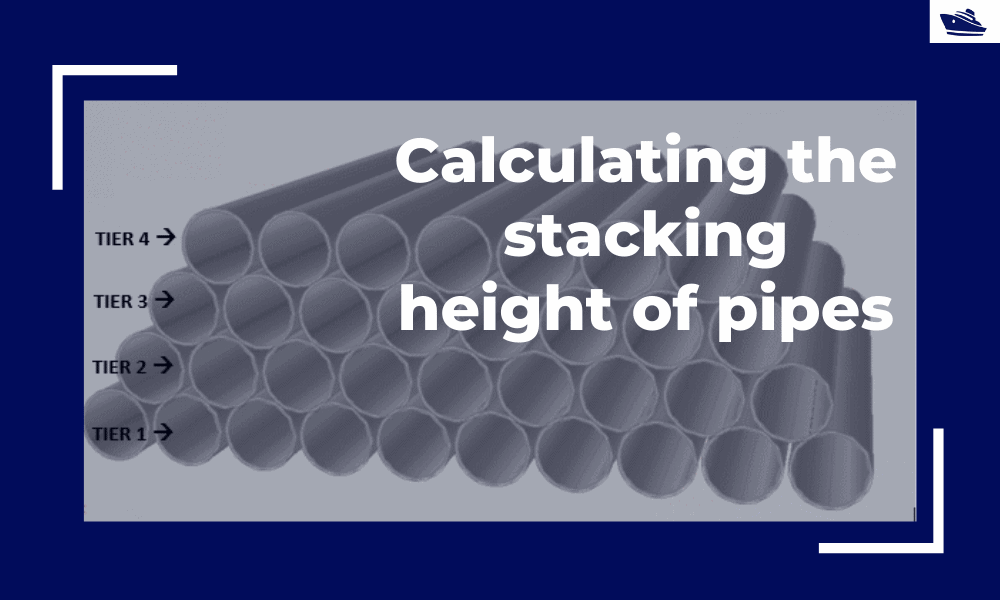## Introduction

Bolts are very commonly used fastening items and used in a variety of configurations. In this article, we will explore in-depth the design of a bolt used in connecting two members at a joint (bolted joint). We’ll see what properties of the bolt are important for its sizing, look into the forces the bolt will experience, and also evaluate the stresses which it will experience.

## Bolted Joint

A bolted joint comprises an incoming member welded to an endplate. The endplate has got bolt holes for connection to another member. We can see this in Figure 1 below.

Figure 1 – Typical joint connection

## Forces on the bolted joint

Any joint will experience the six degrees of freedom – three translations and three rotations. The three translatory movements will result in forces, while the three rotatory movements will result in moments.

Looking at the figure above, we can see that the forces and moments are as below:

• Axial Force in X-direction
• Shear Force in Y-direction
• Sheer Force in Z-direction

The above six forces/moments should be known to the user before commencing the bolt’s design.

## Material Properties of endplate and bolts

The material properties of the endplate and the bolts are the starting inputs for the calculations. For the plate, the yield strength, tensile strength, and material factors are needed.

For the bolts, the bolt grade needs to be selected first. The bolt grades specified in Eurocode 3 are A1, A2, A4, 4.6, 4.8, 5.6, 5.8, 6.8, 8.8, and 10.9. The material properties (yield and tensile strength) will depend on the bolt grade. The material factor for the bolts is user input.

### Bolt Geometry

The geometry of bolts depends on the class of bolt selected. Different classes of the bolt as per the Eurocode 3 are: M10, M12, M16, M20, M24, M27, M30, and M36. Each of them is characterized by the following properties: bolt diameter, bolt hole diameter, and bolt area.

## Plate Geometry

The plate geometry is characterized by the following:

• Endplate thickness tp
• End distances between plate edge and bolt e1 & e2
• Spacings between the bolts in the y and z directions p1 & p2

Figure 2 below illustrated the plate geometry inputs

Figure 2 Plate Geometry Inputs

## Geometry Checks

The spacing of bolts, the end distances from the plate, etc. are subject to some minimum requirements. These are shown below.

p1,p2,e1,e2 are the distances as shown in figure 2, and d0 is the bolt hole diameter, while tp is the plate thickness

With the inputs and geometry check in place, stress assessments of the bolts is the next step.

Figure 3 Bolt Geometry Checks

## Forces and moments

The total forces and moments on the joint are taken by the total number of bolts. The forces and moments on a bolt can be calculated from the forces and moments on the joints. For example, the tensile force on the bolts can be found by dividing the total axial force by the number of bolts, and adding to it the axial forces arising from the moments in the Y and Z directions (by dividing the moments by the lever arm of the moment). Similarly, the shear force can be found out as the resultant of the joint forces in Y and Z directions and adding to it the shear force arising due to the moment in the axial direction.

## Design resistances and capacity checks

The checks for an individual bolt can be performed by calculating the design resistances of a bolt. These include the following and are derived from the material and geometrical properties of the bolt

• Shear resistance
• Tension resistance
• Bearing resistance
• Punching shear resistance

Following the above, the checks for the bolt’s design are performed to calculate the different unity checks as listed below.

• Check in tension
• Check in shear
• Combined shear and tension check
• Bearing Resistance Check
• For end bolts
• For inner bolts
• Punching Shear check

Each of the above checks should result in a UC value of less than 1.0 for the bolt.

That completes this article on designing bolts for joint connections. Please do check out TheNavalArch’s own app on bolt design, and let us know in the comments section if you have any queries.

Disclaimer: This post is not meant to be authoritative writing on the topic presented. thenavalarch bears no responsibility for the accuracy of this article, or for any incidents/losses arising due to the use of the information in this article in any operation. It is recommended to seek professional advice before executing any activity which draws on information mentioned in this post. All the figures, drawings, and pictures are property of thenavalarch except where indicated, and may not be copied or distributed without permission.## Designing a clip/dog plate for seafastening

Introduction In an earlier article, we saw how to design stoppers for seafastening. Stoppers are items that are used to contain the translation movements (longitudinal and transverse directions) of a cargo on the deck/hold of a vessel. That brings us to the question –...## The four important factors for a ship’s windage area calculations

Introduction The windage area of a vessel or offshore structure is the area that is exposed directly to the wind. As is obvious, this is the area of all items above the waterline. This will include Part of the hull/offshore structure above the waterline...## Calculating the maximum stacking height of pipes

Introduction Pipes (or linepipes or joints) are used for multiple purposes and locations in the maritime/offshore industry. Onshore and offshore pipelines are used for transportation of fluids on land, over and underwater. Pipes are fabricated in an onshore facility...